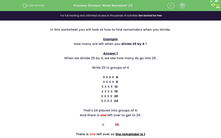# Division: What Remains? (1)

In this worksheet, students learn to find remainders in simple divisions.Key stage:  KS 2

Curriculum topic:   Number: Multiplication and Division

Curriculum subtopic:   Solve Multiplication/Division Problems

Difficulty level:#### Worksheet Overview

In this worksheet you will look at how to find remainders when you divide.

Example

How many are left when you divide 25 by 4 ?

When we divide 25 by 4, we see how many 4s go into 25.

Write 25 in groups of 4

X X X X  4

X X X X  8

X X X X  12

X X X X  16

X X X X  20

X X X X  24

That's 24 placed into groups of 4.

And there is one left over to get to 25.

X            25

There is one left over so the remainder is 1

Another way is to use a number line.

Count up in 4s, until you get to 24.

4    8    12    16    20    24 ...

There is one more to go to get to 25.

The remainder of 1 is shown in blue at the end.### What is EdPlace?

We're your National Curriculum aligned online education content provider helping each child succeed in English, maths and science from year 1 to GCSE. With an EdPlace account you’ll be able to track and measure progress, helping each child achieve their best. We build confidence and attainment by personalising each child’s learning at a level that suits them.

Get started